Copyright © Michael Richmond. This work is licensed under a Creative Commons License.

Elliptical Orbits

So far, we've examined the methods by which several properties of stars can be measured: distance, luminosity, temperature, size. Another fundamental property of a star, or any celestial object, is its mass. How can one measure the mass of a star? It turns out that one must find a star which is in orbit around another star(s) and use gravity as a tool to turn orbital motion into mass.

But it's a complicated subject. Let's start off with a simple example of orbital motion -- planets in our own solar system -- and work our way up to the more complex cases of distant stars.

Kepler's First Law: shape of the orbit

Johannes Kepler was a brilliant mathematician who lived in the late sixteenth and early seventeenth century, a contemporary of Tycho Brahe, Galileo, and Queen Elizabeth I. In order to gain access to the best measurements of planetary motions in the world, he became Tycho Brahe's assistant; his job was not to make the observations, but to analyze them. After Tycho's death in 1601, Kepler spent years working on the great mass of measurements Tycho had acquired.

In 1609, Kepler published a book, Nova Astronomica, in which he made a revolutionary claim: planets don't move in CIRCLES, as everyone had previously thought (including Copernicus); instead,

Kepler's First Law: the planets move in ellipses, with the Sun at one focus.

Let's pause to consider the properties of an ellipse. There are several ways to define this shape:

1. The locus of all points which lie a fixed sum of distances away from two foci.
2. Stick two pins into a piece of paper, and place a loop of string loosely around the pins. Now hold a pen inside the loop and pull outwards until it stretches the string taut. Move the pen around the pins, always keeping the string stretched tightly.
3. In a cartesian coordinate system with axes x and y,4. In a polar coordinate system,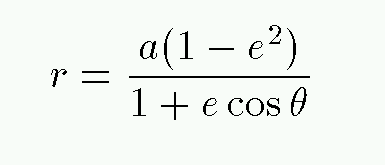Below is a sample ellipse drawn on a background grid. Mark on your piece of paper the following quantities; make all measurements in units of the grid spacing.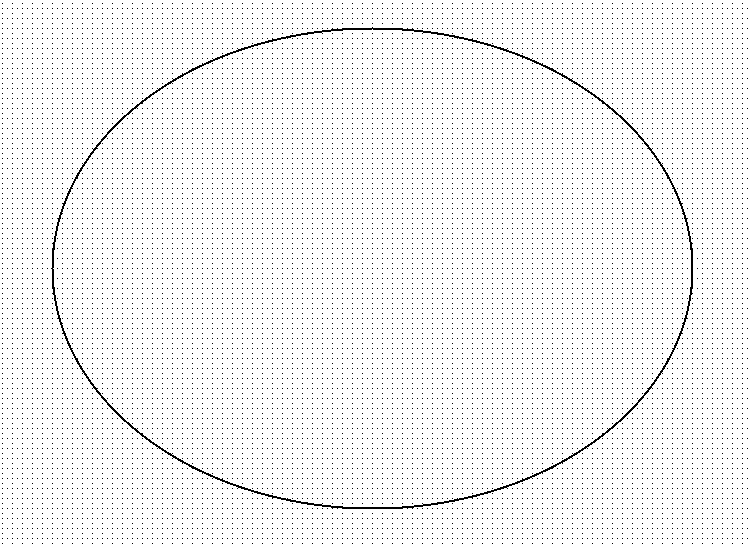1. Measure the semimajor axis a

2. Measure the semiminor axis b

3. Calculate the eccentricity e using the formula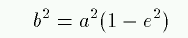4. Calculate the positions of the two foci. Each one lies along the semimajor axis, a distance ae from the center of the ellipse.

5. Mark the two foci. Call the right-hand focus the "principal focus," and draw the Sun at that position.

6. Calculate the perihelion distance and the aphelion distance.

Kepler's Second Law: motion around the orbit

Using Tycho's careful measurements of the position of Mars in our sky, Kepler was able to find a second "imperfection" in the motions of the planets. Not only did they move in ellipses (instead of circles), but their speed was not constant! Instead, the planets move quickly when close to the Sun and slowly when far from the Sun. A nice geometric description is
Kepler's Second Law: A line connecting a planet to the Sun sweeps out equal areas in equal times.

Use this rule on your paper:

1. The planet moves exactly 4 grid units along its orbit at aphelion during time period T. Mark the starting and ending points of this interval on the orbit. Draw the triangle which describes the area swept out during this interval (the apex of the triangle should be the Sun).

2. What is the area of this triangle?

3. Consider a time interval T centered on the planet's passage across the semiminor axis of the orbit. How far along its orbit will the planet move during this interval? Mark the starting and ending points of this interval, and draw the triangle swept out.

4. Consider a time interval T centered on the planet's passage through perihelion. How far along its orbit will the planet move during this interval? Mark the starting and ending points of this interval, and draw the triangle swept out. (Approximate values are okay).

5. What is the ratio of orbital speed at perihelion compared to orbital speed at aphelion for this orbit?

Note that Kepler's Second Law is nice, but it deals only with RELATIVE speeds. We can't use it directly to figure out exactly how far a planet will move over a period of, say, three weeks. Is there any way to figure out exactly where a planet will be at some particular time?

Yes -- two ways, in fact:

• classical approach -- Kepler's equation
• modern approach -- brute-force numerical integration

Thanks to fast, cheap computers, we members of the modern world can simply plug some initial conditions into a computer program and integrate the motion of the bodies numerically to follow their motion as a function of time. One of the problems on this week's homework asks you to do just that.

Back in the old days, however, computing wasn't quite so cheap and easy. Kepler didn't have computers or calculators. In fact, Kepler didn't even have LOGARITHMS; Napier published his first tables in 1632, after Kepler had derived his laws of planetary motion. Kepler did manage to devise a method of computing the position of a planet at any time. It involved a bit of calculating, but nowhere near as much as the modern brute-force approach. Since it's an interesting little mathematical puzzle, let's look at it in some detail.

Kepler's equation for motion around an orbit

The problem is this: we know the orbital parameters of a planet's motion around the Sun: period P, semimajor axis a, eccentricity e. We also know the time T when the planet reaches its perihelion passage. Where will the planet be in its orbit at some later time t?

If the orbit is circular, then this is easy: the fraction of a complete orbit is equal to the fraction of a complete period which has elapsed since the last perihelion passage. For example, if (t - T) is exactly one-quarter of the period P, then the planet will have made exactly one-quarter of a full circle around the Sun.

But, as Kepler's Second Law states, planets in elliptical orbits do NOT move with a constant speed, nor with a constant angular speed. In real life, some planetary orbits are significantly non-circular, so the circular approximation won't work. What can we do?

We can measure the position of a planet in its elliptical orbit with the angle between its radius vector and the perihelion position. This angle is called the true anomaly, and is conventionally written as the letter v.

Yes, I've moved the principal focus closer to the center of the circle than it should be, for clarity.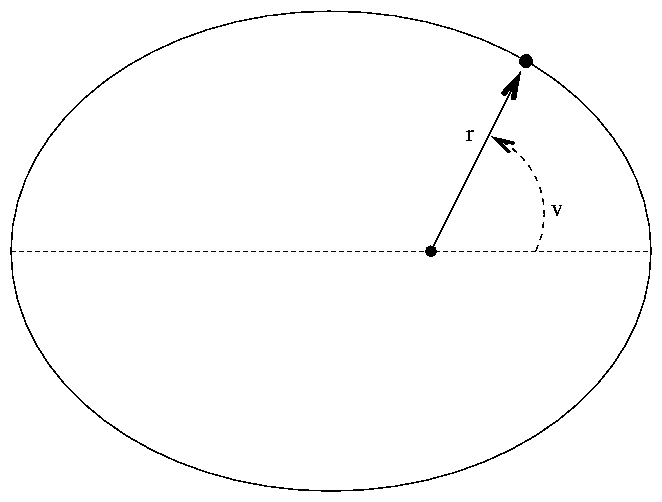What is v as a function of time t?

Kepler found an answer to this question, but it required a bit of a roundabout journey. He discovered that if he defined a "auxiliary" quantity, he could solve for that quantity as a function of time; and then he could convert from the "auxiliary" quantity to the desired true anomaly v.

Kepler's first step was to draw a circle around the ellipse, and project the position of the planet on its elliptical orbit upwards to meet the circle.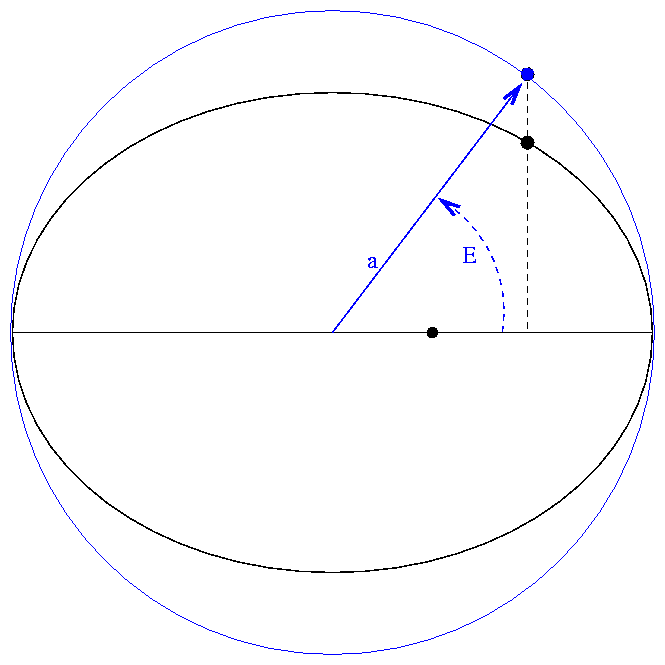The angle E measured from perihelion position, to center of circle, to projected position of planet, is called the eccentric anomaly. If one can find E, one can go back to the desired variables r and v like so: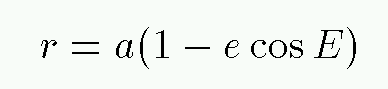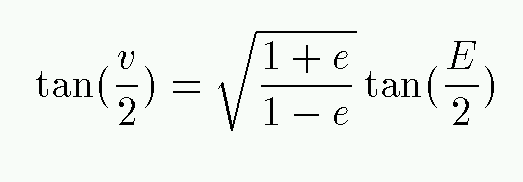Kepler's next step was to find a mathematical relationship between this eccentric anomaly E and time. He computed n, the average angular speed of the planet, also called the mean motion,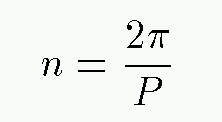You should express the mean motion, and all angular quantities involved in Kepler's equation, in radians.

Finally, he was able to write the following, known as Kepler's equation: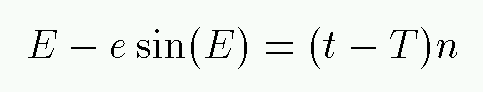Looks good, right? You have to go through several steps:

• find the time of perihelion passage T
• calculate the mean motion n
• solve Kepler's equation for the eccentric anomaly E
• convert to the true anomaly v and radius vector r
but it's not so bad, is it?

Actually, it is. Step 3, solving Kepler's equation for E given some time difference (t - T), turns out to be a doozy. here is no simple closed-form solution, in general, though there are series solutions which converge quickly for small values of the eccentricity. Astronomer Friedrich Bessel devised his eponymous "Bessel Functions" in 1817 as a means to solve Kepler's equation. Letting M = (t - T) n (this is known as the "mean anomaly") one can write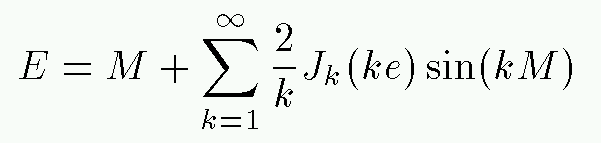where Jk is the k'th Bessel function of the first kind.

Fortunately, even though there isn't a closed solution, Kepler's equation yields pretty quickly to any number of numerical attacks, especially when the eccentricity e is small. You can program a computer to use Newton's method or the the bisection technique. You can even fall back on trial and error. Give it a try.

Exercise: Your piece of paper shows an elliptical orbit. Suppose that the orbit is P = 500 days in a counterclockwise direction. Where will the planet be at (t - T) = 400 days after perihelion passage? Calculate the true anomaly angle v and use it to mark the position of the planet along the orbit.

Start by finding the mean motion n and the mean anomaly M = n(t - T). Use a starting guess that the eccentric anomaly E is equal to the mean anomaly. Plug that E into the left-hand side of Kepler's equation and see what you get. If you get exactly M, you're done! Otherwise, modify your value of E and try again. See if you can find E to four significant figures.Copyright © Michael Richmond. This work is licensed under a Creative Commons License.